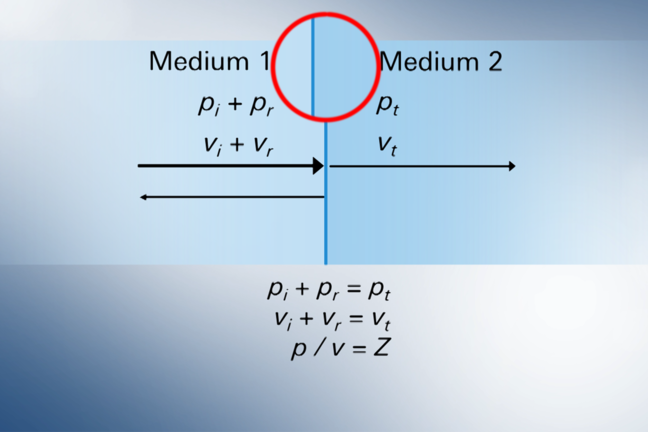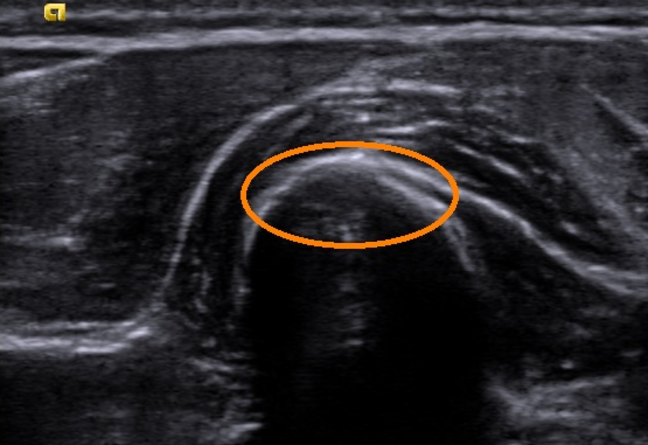## Want to keep learning?

This content is taken from the University of Twente's online course, Ultrasound Imaging: What Is Inside?. Join the course to learn more.
6.17

## University of Twente# Technical bonus - Pressure, velocity, and acoustical impedance

Welcome to the technical bonus article of this course. Although there was not immediately a distinct topic chosen by previous learners in the step dedicated for it, we felt that this topic was not explored very well. Therefore, we try to present you in a more detailed way the relation between sound pressure, velocity, and the acoustic impedance of a medium.

In the video on this topic (see STEP 2.13 - Reflection and transmission) we presented you an example of two media where we looked at the transition of an incoming sound wave at the boundary of two media. To enhance your understanding, it may be helpful to see these media as muscle (Medium 1), and bone (Medium 2).

To describe how much sound is reflected and how much is transmitted we used the term Acoustical impedance (see STEP 2.12 - Acoustic impedance). The equations used here help us to understand the behavior of sound at the boundary between two media. The formulas we have provided you in the video were:

$p_{i}+p_{r}=p_{t}$ $v_{i}+v_{r}=v_{t}$ $p / v = Z$

You may wonder if we shouldn’t have written $$v_{i}-v_{r}=v_{t}$$, as a learner suggested in Week 2 in the comments of our first run. Well, both forms can be correct, depending on how you define positive and negative velocities. We have chosen to define particle motion to the right as positive, no matter whether this is the incoming or the reflected wave. But as an addition, we now must admit that instead of $$p/v=Z$$, we should have written $$p/v=Z$$ for the incoming wave and $$p/v=-Z$$ for the reflected wave. In the video we chose not to do it because it would have made it too lengthy. But it caused confusion for those of you who tried to solve the problem. To see how the relative magnitudes of the reflections and transmissions depend on the acoustical impedances of the two media, you need to solve a system of three equations as explained to you in the video. We will demonstrate this for the ratio $$p_{t} / p_{i}$$.

## Solution

Let’s start with the formulas for pressure and velocity, where we rewrite the velocity formula as function of pressure and acoustical impedance:

$p_{i}+p_{r}=p_{t}$ $v_{i}+v_{r}=v_{t} \Rightarrow \frac{p_{i}}{Z_{1}}-\frac{p_{r}}{Z_{1}}=\frac{p_{t}}{Z_{2}}$

Note that we make use of $$Z_{1}$$ as the acoustical impedance of Medium 1, and $$Z_{2}$$ for Medium 2. Also, we introduced the minus sign since the reflected wave is in opposite direction. Next step is rewriting the second formula so we can add the equations with elimination of $$p_{r}$$:

$\frac{p_{i}}{Z_{1}}-\frac{p_{r}}{Z_{1}}=\frac{p_{t}}{Z_{2}} \Rightarrow p_{i}-p_{r}=p_{t} \frac{Z_{1}}{Z_{2}}$

Placing this next to our initial pressure formula we get the following two equations:

$p_{i}+p_{r}=p_{t}$ $p_{i}-p_{r}=p_{t} \frac{Z_{1}}{Z_{2}}$

Now we make an addition of both complete equations:

$2 p_{i} = p_{t} \left( 1 + \frac{Z_{1}}{Z_{2}} \right)$

Working out of this equation will eventually yield the following:

$2 p_{i} = p_{t} \left( 1 + \frac{Z_{1}}{Z_{2}} \right)$ $\Rightarrow$ $p_{t} = \frac{2 p_{i}}{1+\frac{Z_{1}}{Z_{2}}} = \frac{2 Z_{2} p_{i}}{Z_{1} + Z_{2}}$ $\Rightarrow$ $\frac{p_{t}}{p_{i}} = \frac{2 Z_{2}}{Z_{1} + Z_{2}}$

With this formula, we can see how the sound wave behaves at a boundary of two media. If the media are equal, we have $$Z_{1} = Z_{2}$$. From our formula we can see that the ratio $$p_{t} / p_{i}$$ equals 1, so all the incoming sound waves are transmitted!

When we look at our example of muscle and bone, we have acoustical impedances of $$Z_{1}=1.67\times10^{6}$$ and $$Z_{2}=6.66\times10^{6}$$. When a sound wave travels from muscle to bone, the ratio $$p_{t} / p_{i}$$ equals $$\frac{2*6.66}{1.67+6.66}=1.60$$. This means that the pressure amplitude of the transmitted sound wave is about 60% higher than the pressure of the incoming wave.

The same can be done if you want to find the ratio between pressure of the reflected and the incoming wave. If you follow the same steps and method to solve the system of three equations, you will finally obtain the formula for the ratio between the pressure of the reflected wave and the incoming wave. From this formula, you get that $$p_{r} / p_{i} = \frac{Z_{2}-Z_{1}}{Z_{1}+Z_{2}}=0.6$$. This means that a large part of the sound wave will be reflected in our example of muscle and bone. The reflection at the bone surface can thus be well observed as a bright surface, which can be seen in the orange circle in the image below.We ask you now to perform the following tasks. You can place your ideas or solutions in the comment section below. Please try not to start with reading other learners’ comments, but try it yourself first. Our tasks/questions are:

1. Show how the formula for the ratio of the reflected wave and the incoming wave as a function of acoustical impedances can be derived from the system of three equations.
2. Use the formulas to calculate the ratios $$\frac{p_{t}}{p_{i}}$$ and $$\frac{p_{r}}{p_{i}}$$ for the transitions water-muscle, air-fat, and fat-muscle using the table of Acoustic impedances (see STEP 2.12 - Acoustic impedance).